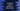# JavaScript program to find the sum of all odd numbers below one given number# JavaScript: find the sum of all odd numbers below one given number :

To find the sum of all odd numbers less than one specific number, we need to check if a number is odd or not for all numbers less than that number. If odd is found, we will add it to a final sum variable. This sum variable will hold the total sum of all numbers and it will be initialized as 0.

## Javascript program :

The javascript program to find the sum of all odd numbers less than a given number looks like as below :

``````function isOdd(n) {
return Boolean(n % 2);
}

function findSum(no) {
let sum = 0;

for (var i = 0; i < no; i++) {
if (isOdd(i)) {
sum += i;
}
}
return sum;
}

console.log(findSum(100));``````

### Explanation :

1. We have two functions here. findSum and isOdd.
2. findSum is the main function to find the sum. It takes one number and returns the sum.
3. isOdd takes one number and returns one Boolean value based on the number is divisible by 2 or not.
4. sum variable is used to hold the sum of all odd numbers.
5. One for loop runs from i = 0 to i < no and checks for each number if it is odd or not. isOdd is used to check for that.
6. If the current number is odd, this number is added to sum.
7. Finally, the value of sum is returned from findSum

In this example, we are finding out the sum of all odd numbers less than 100, but we can use this same program to find out for any user given number.